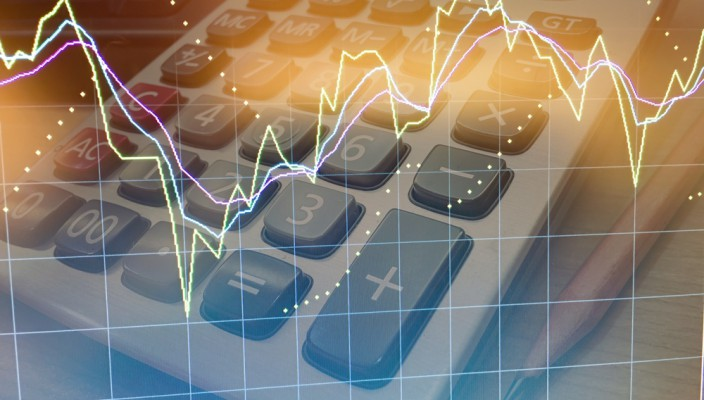## Fibonacciand its Application to Forex Trading

Feb 22 • Forex Trading Articles • 4363 Views • Comments Off on Fibonacciand its Application to Forex Trading

Of all the: terms, patterns, indicators and tools used in trading, the word, allure and concept of “Fibonacci” stands out as the most mysterious and evocative. It’s legendary use in mathematical calculus, lends it an authority not associated with the modern, most commonly used chart indicators, such as the: MACD, RSI, PSAR, DMI etc.

It may surprise many novice traders to learn that the ‘original’ Fibonacci sequence is used by many traders and quants at major institutions when designing algorithmic trading models, in their attempts to take profit out of the market. A brief history lesson on Fibonacci is appropriate at this point, before we venture into how we can use this pure, mathematical, phenomenon on our charts.

The Fibonacci sequence was named after the Italian mathematician Leonardo of Pisa, known as Fibonacci. His 1202 book Liber Abaci introduced the phenomenon to European mathematics. The sequence had been described earlier as Virahanka numbers in Indian mathematics.

Fibonacci explained his theory by using the growth example of a (theoretical) rabbit population, a newly born pair of rabbits mating at the age of one month. At the end of its second month a female can produce another pair of rabbits, the assumption is the rabbits never die, the mating pair produce one new pair (one male, one female) every month from the second month on. The puzzle that Fibonacci posed was: how many pairs will there be in one year? The mathematical model explaining this expansion became the Fibonacci sequence. The number sequence appears in biological settings: branches in trees, leaves on a stem, fruit sprouts of a pineapple,artichoke flowering, uncurling ferns and pine cones’ bracts.

So how does this mathematical sequence, discovered and developed over 800 years back, have relevance to modern day forex trading? There are two beliefs where the application is concerned. One concerns what’s termed a “self fulfilling prophecy”. The other application relates to a supposed natural contraction in sentiment, as the energy of a movement dissipates; a sharp market movement will then retrace to certain levels. Let’s deal with the self fulfilling theory before we explain the maths behind the retracement theory.

The self fulfilling theory suggests that if many traders are using the Fibonacci retracement theory, then the market has the possibility to revert to these levels and there has been evidence to prove this theory may often be at work in the markets. If enough traders at: major banks, institutions, hedge funds and enough designers of algorithmic trading methods, use the retracement sequence to place orders, then the levels may be hit. The key danger is that whenever we experience a significant surge on, for example, a major currency pair, the chance exists that we’ll experience a significant retraction, for a variety of reasons. As price falls back many Fibonacci fans will claim “eureka! It’s worked again!” When the reality could be the market participants simply overbought or oversold the market and are now experiencing doubts, whilst the market pauses to find a new ‘natural’ level.

Now let’s look at how the wave of sentiment may retract and the maths come into play. You start by simply finding the top and bottom of the market move and plot the two points, this is 100% of the move. The most commonly used Fibonacci levels are the 38.2%, 50%, 61.8%, sometimes 23.6% and 76.4% is used, although the 50% level is not actually part of the maths sequence, it’s been inserted over the years by traders en masse. In a strong trend a minimum retracement is circa 38.2%, in a weak trend, the retracement could be 61.8% or 76.4%. A complete retracement (of 100% of the move) would eradicate the existing move.

Fibonacci levels should only be calculated after a market has made a large move and appears to have flattened out at a certain price level. If not automatically calculated by the charting package,  the Fibonacci retracement levels of 38.2%, 50% and 61.8% are set by drawing horizontal lines oncharts to identify areas where the market may retrace to, before resuming the trend originally formed by the initial large price move. What follows now are a few strategies forex traders use for trading Fibonacci levels.

•  Enteringclose to the 38.2% retracement level, stop loss just below the 50% level.
•  Enteringclose to the 50% level, stop loss order just below the 61.8% level.
•  Shorting near the top of the move, using Fibonacci levels as take profit targets.

As always it’s up to traders to practice using Fibonacci. A good place to start would be back/testing by plotting the tops of bottoms on a daily chart. Simply find the key large movements, find the peak and trough and establish if the retracement actually ‘worked’. Similar to all trading methods none are absolute, none are 100% reliable. However, we’ve all witnessed, time after time, our markets recoil and retrace after a large market movement. If you can then attach some maths and science to that retrace and underpin it with (you’ve guessed it), a sound money management technique, then you may discover that adding Fibonacci into your trading strategy works extremely well.